# Liquid Flow in Pipes

| Home | | Pharmaceutical Technology |

## Chapter: Pharmaceutical Engineering: Fluid Flow

The many pharmaceutical processes that involve the transfer of a liquid confer great importance on the study of flow in pipes.

LIQUID FLOW IN PIPES

The many pharmaceutical processes that involve the transfer of a liquid confer great importance on the study of flow in pipes. This study permits the evalu-ation of pressure loss due to friction in a simple pipe and assesses the additional effects of pipe roughness, changes in diameter, bends, exists, and entrances. When the total pressure drop due to friction is known for the system, the equivalent head can be derived and the power requirement for driving a liquid through the system can be calculated from equation (2.14).

Streamline Flow in a Tube

The mathematical analysis of streamline flow in a simple tube results in the expression known as Poiseuille’s law, one form of which is equation (2.14):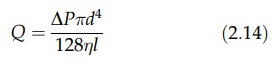where Q is the volumetric flow rate or discharge, ΔP is the pressure drop across the tube, d and l are the diameter and length of the tube, respectively, and η is the viscosity of the fluid.

Whether flow in the tube is streamline or turbulent, an infinitesimally thin stationary layer is found at the wall. The velocity increases from zero at this point to a maximum at the axis of the tube. The velocity profile of streamline flow is shown in Figure 2.9A. The velocity gradient du/dr is seen to vary from a maximum at the wall to zero at the axis. In flow through a tube, the rate of shear is equal to the velocity gradient, and equation (2.1) dictates the same variation of shear stress.FIGURE 2.9 Streamline flow. (A) Velocity profile in a pipe; (B) longitudinal terms and (C) cross-sectional terms used to define flow properties.

To derive Poiseuille’s law, the form of the velocity profile must first be established. For a fluid contained within a radius r flowing in a tube of radius R, this is shown in Figure 2.9B. If the pressure drop across length l is ΔP, the force attributed to the applied pressure driving this section is ΔPpr2. If the flow is steady, this force can only be balanced by opposing viscous forces acting on the “wall” of the section. This force, the product of the shear stress t and the area over which it acts, is 2tπl. The expression given by equating these forces is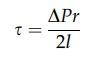Substituting from equation (2.1) gives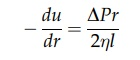The velocity gradient is negative because u decreases as r increases. When r = R, u = 0. Integration gives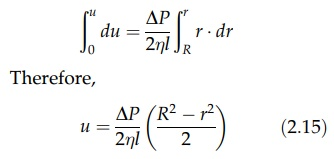This relation shows that the velocity distribution across the tube is parabolic. For such a distribution, the maximum velocity is twice the mean velocity. The volumetric flow rate across an annular section between r and (r + dr) shown in Figure 2.9C is

Q = 2πr.dr. r

Substituting for u from equation (2.15) givesThe total volumetric flow rate is the integral between the limits r = R and r = 0: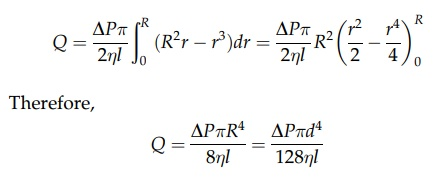which is equation (2.14), where d is the diameter of the tube. Since Q = umeanp(d2/ 4), substitution and rearrangement givesDimensional Analysis and Flow Through a Tube: A General Approach

The utility of equation (2.16) for evaluating the loss of pressure due to friction in a tube is limited because streamline conditions are rare in practice. The theo-retical analysis of turbulent flow, however, is incomplete, and experiments with fluids are necessary for the development of satisfactory relations between the controlling variables, one in terms of the other, while other variables are tem-porarily held constant. Dimensional analysis is a procedure in which the interaction of variables is presented in such a way that the effect of each variable can be assessed.

The method is based on the requirement that the dimensions of all terms of a physically meaningful equation are the same, that is, an equation must be dimensionally homogeneous. This principle may be usefully illustrated by ref-erence to equation (2.14) written in the form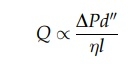Reviewing in basic units of mass, length, and time and using the symbol [ ] to represent dimension of [Q] = [L3T-1], [ΔP] = [ML-1T-2], [dn] = [Ln], and [η] = [M L-1T-1], equating gives[M] and [T] are correct, as they must be. Equating [L] gives [L3] = [Ln-1] from which n = 4.

If no previous knowledge of the combined form of the variable that determines Q is available, dimensional analysis can be applied in the following way. The dependence of Q on ΔP, l, d, and η can be written as

Q = fP; l; d; η)

The function f can be expressed as a series, each term of which is the product of the independent variables raised to suitable powers. Taking the first term of the series gives

Q = N . ΔPw . Lx . Dy . ηz

where N is a numerical factor (dimensionless). Rewriting terms as [Q] = [L3T-1], [ΔPw] = [MwL-wT-2w], [lx] = [Lx], [dy] = [Ly], and [ηz] = [MzL-zT-z], the equation [Q] = [ΔPw·lx·Dy·ηz] becomes [L3T-1] = [MwL-wT-2w·Lx·Ly·MzLzT-z].

Equating powers of M, L, and T gives the following system:

M:0 = w + z

L : 3 = -w + x + y - z

T: - 1 = - 2w - z

Since four unknowns are present in three simultaneous equations, three may be determined in terms of the fourth. Solving gives w = l, z = -1, and x + y = 3.

Expressing y as 3’ - x, one gets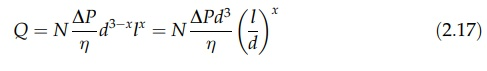The first part of the example demonstrates the use of dimensions as a partial check on the derivation or completeness of a solution. In the second part, a solution, although incomplete, gives considerable information about discharge of a fluid in streamline flow and its relation to pressure drop, viscosity, and the geometry of the pipe without any theoretical or experimental analysis. For example, if two tubes had the same ratio l/d, the values of Q/ηd3ΔP would also be the same.

Since the exponent x in equation (2.17) is indeterminate, the term in brackets must be dimensionless. Unlike the lengths from which it is derived, it is a pure number and needs no system of units for meaningful expression. Its value is, therefore, independent of the units chosen for its measurement, provided, of course, that the systems of measurement are not mixed. The equation may, therefore, be presented as the relation between two dimensionless groups as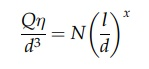or, since a series of power terms will, in general, form the original unknown function, each of which has different values of N and x,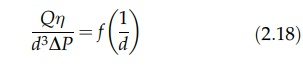The study of frictional losses at the wall of a pipe is facilitated by dimensional analysis. The shear stress—that is, the force opposing motion of the fluid acting on each unit of area of the pipe, R—is determined for a given pipe surface by the velocity of the fluid, u, the diameter of pipe, d, the viscosity of the fluid, η, and the fluid density, ρ. The equation of dimensions is

[R] = [uo dq  ηy ρs]

Therefore,

ML-1T-2 =  LpT-p . Lq . M1L-rT-r . MsL-3s

Equating M, L, and T, one gets

M: 1 = r + s

L: -1 = p + q - r - 3s

T: -2 = - p - r

Solving for p, r, and s in terms of q gives r = -q, s = 1 + q, and p = 2 + q.

Therefore,where N is a numerical factor. Generalizing, R/ρu2, which is the friction factor, is a function of a dimensionless combination of u, d, η, and ρ. This combination gives a parameter known as the Reynolds number, Re. Therefore,FIGURE 2.10 Pipe friction chart: R/ρμ2 versus Reynolds number.

In turbulent flow, the shear stress at the wall depends on the surface, the value being higher for a rough pipe than for a smooth pipe when flow conditions are otherwise the same. Equation (2.19) therefore yields a family of curves when pipes are of differing dimensionless group, e/a, in which e is a linear dimension expressing roughness. Values of e are known for many materials.

The complete dimensionless correlation, plotted on logarithmic coor-dinates so that widely varying conditions are covered, is given in Figure 2.10. The curve can be divided into four regions. When Re < 2000, flow is streamline and the equation of the line in this region is R/ρu2 = 8/Re. This is simply another form of Poiseuille’s law. The friction factor is independent of the roughness of the pipe, and all data fall on a single line.

When Re lies between 2000 and 3000, flow normally becomes turbulent. The exact value of the transition depends on the idiosyncrasies of the system. For example, in a smooth pipe, streamline conditions will persist at higher Reynolds number than in a pipe in which disturbances are created by surface roughness.

At higher values of Re, flow becomes increasingly turbulent to give a region in which the friction factor is a function of Re and surface roughness. Ultimately, this merges with a region in which the friction factor is independent of Re. Flow is fully turbulent, and for a given surface, the shear stress at the pipe wall is proportional to the square of the fluid velocity. The onset of the fourth region occurs at a lower Re in rough pipes.

The essential difference between laminar flow and turbulent flow has already been described. In a pipe, the enhanced momentum transfer of the latter modifies the velocity distribution. In laminar flow, this distribution is parabolic. In turbulent flow, a much greater equalization of velocity occurs, the velocity profile becomes flatter, and high-velocity gradients are confined to a region quite close to the wall. In both cases, the boundary layer, the region in which flow is perturbed by the presence of the boundary, extends to the pipe axis and completely fills the tube. In laminar conditions, the structure of the layer is quite simple, layers of fluid sliding one over another in an orderly fashion. In tur-bulent flow, however, division can be made into three regimes: (i) the core of fluid, which is turbulent; (ii) a thin layer at the wall, which is a millimeter thick and where laminar conditions persist; this is called the laminar sublayer, and it is separated from the turbulent core by (iii) a buffer layer in which transition from turbulent flow to laminar flow occurs.

This description of the turbulent boundary layer applies generally to the flow of fluids over surfaces. The properties of this layer are central in many aspects of the flow of fluids. In addition, these properties determine the rate at which heat or mass is transferred to or from the boundary. These subjects are described in chapters 3 and 4.